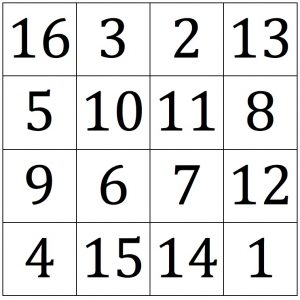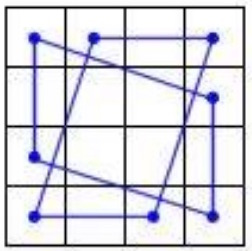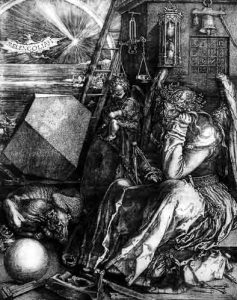Add the columns one by one, add the rows, then add the diagonals. What do you notice?Add the numbers in the squares at the corners of each parallelogram shown. What do you notice? Yes, so far all these totals have been 34.

In addition the diagram with the two parallelograms has 4-fold rotational symmetry so there is a lot of mathematics in this supermagic square.This is Albrecht Durer’s supermagic square from his famous engraving Melencolia dated 1514. Can you see it at the top right hand corner of the engraving under the bell? It has many more special properties.

Now investigate the totals of different sets of 4 numbers chosen from this magic square and draw diagrams like the one given above. Can you find 10 diagrams and, for each one, describe the quadrilaterals you draw and describe the symmetries in the diagram.

A friend visited a school in Denmark and photographed this seat in the playground.

What is the same and what is different about the magic square on the seat from Durer’s magic square?